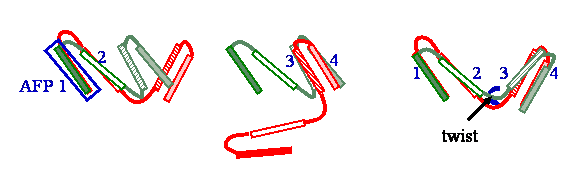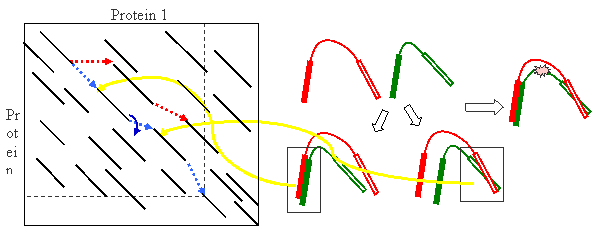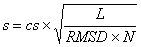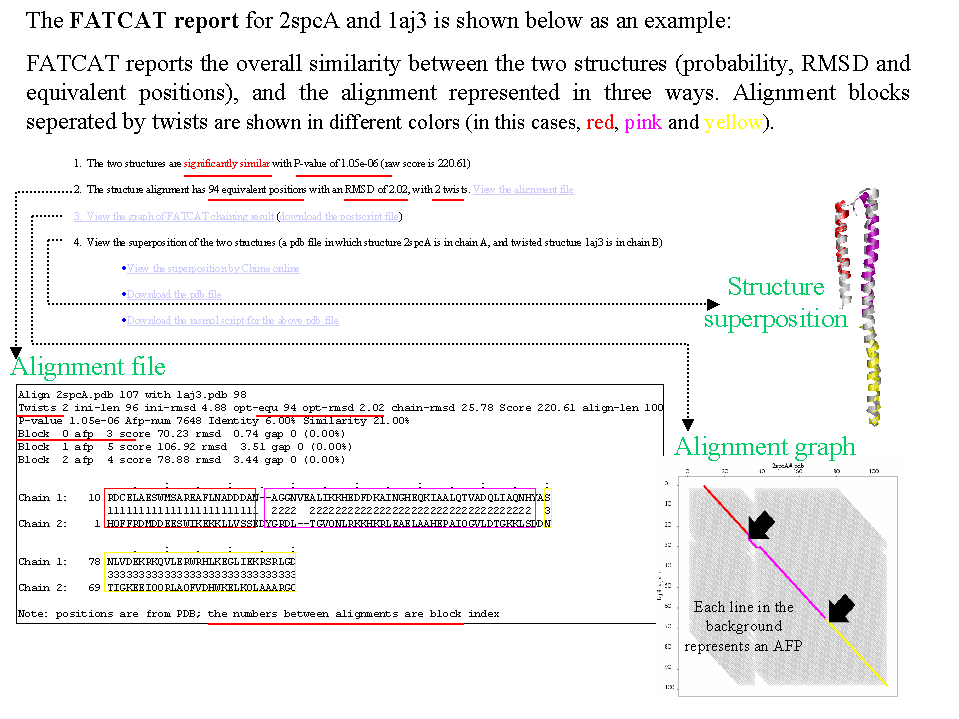## AFP (Aligned Fragment Pair)

Given two protein structures, denote a match of two fragments (i.e., length = 8 aa), one from each protein as an Aligned Fragment Pair (AFP). Each AFP can define a transformation of two structures. The figure below shows two AFPs, which define two very different transformations of input structures.## Twist

As shown in the schematic example below, the green structure has to be rearranged (a twist introduced at the hinge, pointed by an arrow) so that the green and red structures can be better aligned (i.e., including 1-4 AFPs, instead of only two, either 1-2 or 3-4).## FATCAT (chaining) score

In FATCAT, Flexible structure alignment is formulated as an AFP chaining process (e.g., the path connected by blue dotted lines in the alignment graph below represents a possibe alignment) allowing at most t twists (t=5). Dynamic programming is used in the chaining process (as shown in the figure below). If we denote S(k) as the best score ending at AFP k, it can be calculated from the best ending at previous AFPs that can be connected with AFP k subject to the constraints of the consecutive,where a(k) is the score of AFP k itself, determined by its RMSD (dk) and length (L) with long AFPs rewarded and large RMSDs penalized;is the score of introducing a connection between AFP m and AFP k, defined by a function of the compatibility of the AFPs and the mis-matched regions (p) and/or gaps (q) created by the connection of the two AFPs; T(k) is the number of twists required to connect the chain of AFPs leading up to S(k).

The FATCAT (chaining) score is the best of all S(k) in the alignment graph.## P-value

P-value is used in FATCAT to evaluate the significance of structural similarity detected by FATCAT, the probability of observing a greater score. It was designed based on the observation that the FATCAT similarity score between two unrelated structures follows the extreme value distribution. Briefly, FATCAT similarity score incorporates the FATCAT chaining score, RMSD of the resulting superposition, the number of equivalent positions in the alignment and the number of twists.

The FATCAT similarity score is computed aswhere cs is the FATCAT chaining score; L is the number of equivalent positions in the alignment; RMSD is the overall RMSD between two structures when one structure is rearranged at the positions where twists are detected by FATCAT; N is the number of blocks in the alignment (number of twists + 1).

P-value of s is then computed aswhere the location and the scale parameter of the EVD of FATCAT similarity scores of random structures were determined by empirical simulation.

## align-len

The length of the alignment (including gaps)

## opt-len

The number of equivalent positions of the alignment
opt_len = align_len - gap

## opt-rmsd

The root mean square deviation (RMSD) of aligned Cα atoms of the input structures, with one input structure rearranged if flexibility is detected (i.e., twists are introduced in the alignment)

## chain-rmsd

The root mean square deviation (RMSD) of aligned Cα atoms of the input structures, without structural rearrangement even structural flexibility is detected in the alignment. So in the cases with flexibility (i.e., twists are introduced to get the alignment), the value of chain-rmsd could be artifically very high (because flexible alignment is longer than rigid alignment). Yet the comparison of chain-rmsd and opt-rmsd provides a way of showing how signifcantly the conformational flexibility introduced in comparing the structures improves the alignment.